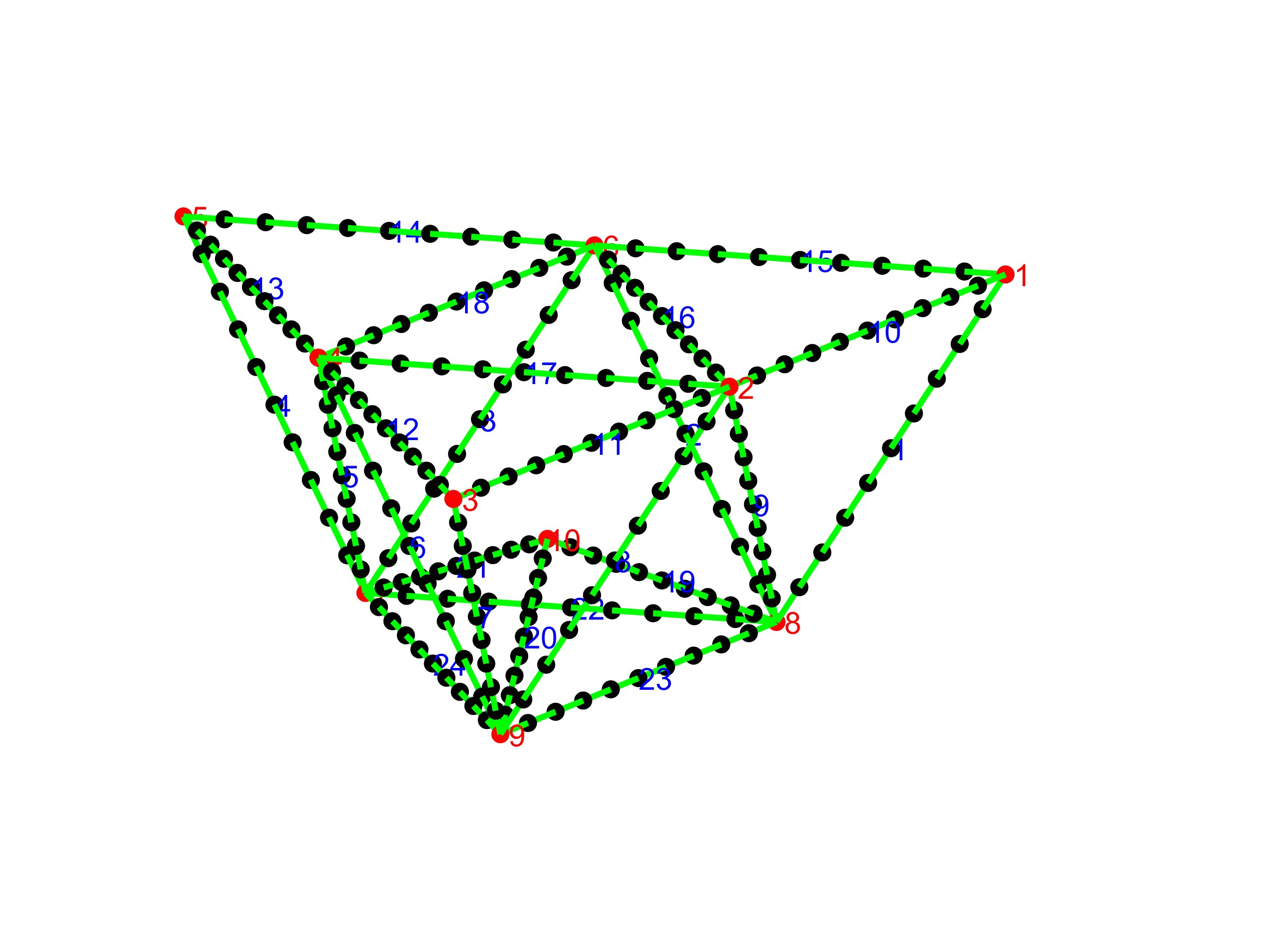## Beam Truss

Version 1.0 (21.1 KB) by
A MATLAB code to generate the mass and stiffnes matrices for a beam truss structure and conduct a modal analysis. The beam model is based on

Updated 17 Mar 2023

From GitHub

Beam Truss Model
A MATLAB code to generate the mass and stiffnes matrices for a beam truss structure and conduct a modal analysis. The beam model is based on the Euler-Bernoulli assumptions.
Features
• generation of mass and stiffness matrices
• modal analysis
• geometry plot
• dynamic visualization of the modes in a video
• additional inertia of connection elements is accounted for
How to use
1. Setup
Specify the beam truss using the matrices P and Beam.
The rows of P are the connection points between the beams. The first column numbers the connecton points, the second until fourth column describe the x- y- and z-coordinate.
for example
P= [1 0 -0.6351 0.2935,
2 -0.2750 -0.1588 0.2935,
...
10 0 0 0];
The rows of Beam each stand for a beam of the truss. The beams are numbered in the first column. The second and third column specify the number of the start and end connection point of the respective beam. The numbers of the connection points had been previously defined in P.
for example
Beam = [1, 8, 1
2, 6, 8
...
24, 7, 9];
Generate an instance of the beam_truss class. Specifiy the number n of elements per beam.
my_truss = beam_truss(P,Beam,n);
The geometry is plotted subsequently.2. Modal Analsysis
Conduct a modal analysis using the command
modal_analysis(my_truss)
and visualize a selected mode i_mode
disp_modal_analysis(my truss, i_mode)
Please note that by default only the first 35 modes are calculated, but that can be changed easily in the code so you can also look into higher-order modes. Since the structure is not fixed, the first six eigenmodes will be rigid body modes.
Troubleshooting
The code should be fairly well documented. If you find a bug, please let me know and I will look into it.
Literature
 Géradin, Michel, and Daniel J. Rixen. Mechanical vibrations: theory and application to structural dynamics. John Wiley & Sons, 2014. (highly recommended)
 Shabana, Ahmed A. Dynamics of multibody systems. Cambridge university press, 2020.
##### MATLAB Release Compatibility
Created with R2023a
Compatible with any release
##### Platform Compatibility
Windows macOS Linux